Definitions of Square Dance Calls and Concepts

Index -->  Plus  |  A1  |  A2  |  C1  |  C2  |  C3A  |  C3B  |  C4  |  NOL  |
Definitions (Text Only) -->  Plus  |  A1  |  A2  |  C1  |  C2  |  C3A  |  C3B  |  C4  |  NOL  |
 Find call:

Divide The Ocean | Sea direction | anything -- [C3B]
(Lee Kopman 1979)

\$B0lHL2=\$5\$l\$?(B Tidal Line \$B\$+\$i(B.

Outside 4 \$B\$O(B Cast Off 3/4 \$B\$r\$7(B Cross Concentric Vertical 1/2 Tag (Ocean \$B\$N\$H\$-(B) \$B\$^\$?\$O(B Cross Concentric Vertical Left 1/2 Tag (Sea \$B\$N\$H\$-(B) \$B\$r\$7(B, \$BB>\$N?M\$O(B Hinge, Partner Tag, Press Ahead, \$B\$=\$7\$FM?\$(\$i\$l\$?(B direction \$B\$NJ}\$X(B 1 \$B?M\$G8~\$-\$rJQ\$(\$k\$+(B anything \$B\$N%3!<%k\$r9T\$\$\$^\$9(B.

Divide The Sea Out: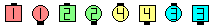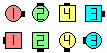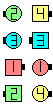Divide The Sea Out\$B\$NA0(B Outsides Cast Off 3/4 as Centers Hinge & Partner Tag\$B\$N8e(B Outsides Cross ConcentricVertical Left 1/2 Tag as Centers Press Ahead & 1/4 Out\$B\$N8e(B (\$B=*\$o\$j(B)

\$BCm
• New Outsides \$B\$,(B anything \$B%3!<%k\$r(B Concentric \$B\$G9T\$\$\$?\$\$\$H\$-\$O(B, \$B%3!<%i!<\$O\$=\$N\$h\$&\$K(B (\$BNc\$(\$P(B, Tidal Wave \$B\$+\$i(B: Divide The Ocean Concentric Shakedown) \$B;X<(\$7\$J\$1\$l\$P\$J\$j\$^\$;\$s(B.
• Direction \$B\$d(B anything \$B%3!<%k\$N%G%U%)%k%H\$O\$"\$j\$^\$;\$s(B. \$B%3!<%i!<\$,(B direction | anything \$B\$r8@\$o\$J\$+\$C\$?\$H\$-\$O(B, \$B%*%j%8%J%k\$N(B Centers \$B\$O(B Hinge, Partner Tag, Press Ahead \$B\$^\$G\$r\$7\$^\$9(B. \$BLuJN,\$5\$l\$?\$H\$-\$K;H\$o\$l\$kCM\$r;X\$98@MU\$G(B, \$B!V(BSquare Thru \$B\$N%G%U%)%k%H\$O(B 4\$B!W\$N\$h\$&\$K;H\$\$\$^\$9(B.
• Single Divide The Ocean | Sea direction | anything [C4]: \$B0lHL2=\$5\$l\$?(B Line \$B\$+\$i(B. Ends \$B\$O(B 3/4 Out (Single Cast Off 3/4) \$B\$r\$7(B, Step Ahead \$B\$r\$7(B Touch (Ocean \$B\$N\$H\$-(B) \$B\$^\$?\$O(B Left Touch (Sea \$B\$N\$H\$-(B) \$B\$r\$7(B, Centers \$B\$O(B Hinge, Partner Tag, Press Ahead \$B\$r\$7(B, \$BM?\$(\$i\$l\$?(B direction \$B\$X(B 1 \$B?M\$G8~\$-\$rJQ\$(\$k\$+(B anything \$B\$N%3!<%k\$r9T\$\$\$^\$9(B. \$BIaDL(B Line \$B\$G=*\$o\$j\$^\$9(B.

Single Divide The Ocean Right: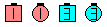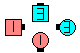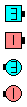Single Divide The Ocean Right\$B\$NA0(B Outsides Single Cast Off 3/4 as Centers Hinge & Partner Tag\$B\$N8e(B Outsides Cross Concentric Touch as Centers Step Ahead & 1/4 Right\$B\$N8e(B (\$B=*\$o\$j(B)Choreography for Divide The Ocean | Sea direction | anythingComments? Questions? Suggestions?

https://www.ceder.net/def/dividetheocean.php?level=C3B&language=japan
26-January-2021 11:25:17